# 10 Compound Interest Examples and a Free SpreadsheetThese 10 compound interest examples will take you from the basic calculations to where the magic happens.

I first learned about compound interest in my high school economics class, and it immediately clicked.

Earning interest on interest seemed like an obvious path to grow wealth.

Why wasn’t everybody doing this?

The 10 compound interest examples in this article give you the basic to intermediate knowledge to utilize compound interest to grow your wealth.

## What is Compound Interest?

Think of compound is as earning interest upon interest, or as Merriam-Webster puts it:

Interest computed on the sum of an original principal and accrued interest.

Here’s a simple example that we’ll build on for the rest of the article.

If an investor puts \$1,000 into an investment that earns 5% annually, that investment will pay \$50 in year 1.

At the start of year 2, the investor has \$1,050.00. She earns 5% on \$1,050, or \$52.5.

At the start of year 3, the investor has \$1,102.50. She earns 5% on \$1102.50, or \$55.13.

At the start of year 4, the investor has \$1,157.63. She earns 5% on 1,157.63 or \$57.88.

After four years, the investor has \$1,215.51.

And so on.

The calculation is the same whether you start with \$0.01 or \$1 billion. But it only compounds if you reinvest the earnings (or hold for capital appreciation).

Spending the earnings will prevent the compounding from occurring. You have to let it ride.

## Why Compound Interest is Important

All of the examples in today’s post and the spreadsheet are overly simplified to demonstrate how compound interest works.

For the first example above, think of it as an interest-bearing savings account (if you can dream of one paying 5%).

In the real world, scenarios are far more complicated because investment values fluctuate by the second, and our savings and new investments change based on income and spending.

To calculate compound interest for a stock portfolio, we use the compound annual growth rate (CAGR), which is:

A hypothetical constant interest rate that would be required for compound interest to turn a given present value into a given future value in a given amount of time.

Calculating an investment portfolio’s future value allows us to estimate our retirement date and future financial needs. It’s critical to financial planners and DIY investors.

Compound interest is what will ultimately make most of us wealthy, not our careers.

## The Compound Interest Formula

We’ll use basic math to demonstrate compound interest first. If this gives you scary high school flashbacks, skip to the next section for the spreadsheet version.

Here is the basic compound interest formula. It solves for the accrued amount, aka, future value

`A = P*(1 + r/n)^nt`

Where:
A = the accrued amount
P = the initial principal
r = interest rate (expressed as a decimal)
n = number of compoundings per year
t = total number of years (time)

Using the same numbers from the previous example above, it looks like this, solving for A:

```A = 1000*(1 + (.05/1))^(1*4)
A = 1000*(1.05)^(4)
A = 1000*(1.05)^4
A = 1000*(1.215506)
A = 1215.51```

OK, enough math class. We’ll use a spreadsheet from now on.

## The Free Compound Interest Spreadsheet

This article steps you through ten calculations, starting with the compound interest formula, then building a series of compound interest examples to demonstrate variations.

I use a 4-year time period for the first group to explain the calculations, then expand to a 40-year horizon and add a few charts to show the magic of compound interest.

Having a spreadsheet will help you understand each calculation that I’ve screen-captured, and let you play with the numbers and experiment with your own scenarios.

Use the parameters on the left to input your numbers.

## Compound Interest Examples

If the above compound interest formula looks familiar to some of you, it’s the Future Value (FV) function in Excel. Those of us who studied Finance in college are all too familiar with it.

Here’s the FV excel function, continuing with the previous example:

`=FV(0.05,4,0,-1000)`

Type or paste that into a spreadsheet, and you’ll get the same result: \$1,215.51

I rarely use the Excel financial functions because I like to break the calculations out by period.

Here’s how I calculate compound interest in a spreadsheet using the same values. From here on, I set aside the parameters on the left side and build the table from the parameters.

You can play with the parameters as you please.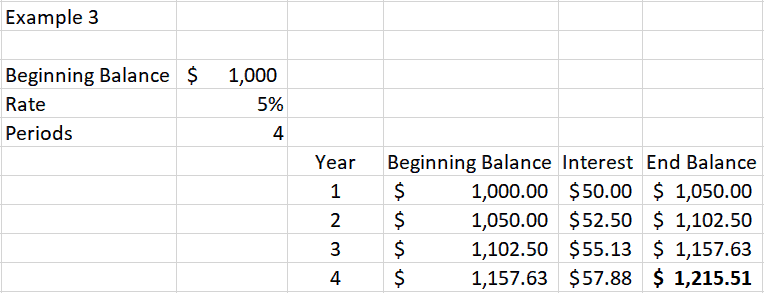### Variable Compounding

We’ve kept things very simple so far, using a fixed starting principal amount, annual compounding, and no regular contributions (next section).

When the compounding occurs more frequently, the Future Value of an investment increases, because the interest paid adds to the principal sooner, earning more interest along the way.

If you’ve heard of the term front-loading retirement accounts (contributing the IRA max in early January), this explains why the practice optimizes returns.

Compounding frequency varies depending on the investment. CAGR is annual, high-yield savings accounts compound daily, dividends are quarterly or monthly, and bonds usually pay out semiannually (and you must reinvest the interest to get the compounding effect).

Next, we’ll look at how to calculate compound interest at different frequencies for the same above example to see how it changes the outcome.

#### Quarterly Compounding

Over the same 4-year period, if we choose to compound the initial \$1,000 investment quarterly, or 16 times instead of four times over four years, we end up with \$1,219.89. That’s a few dollars higher than the annual compound interest example.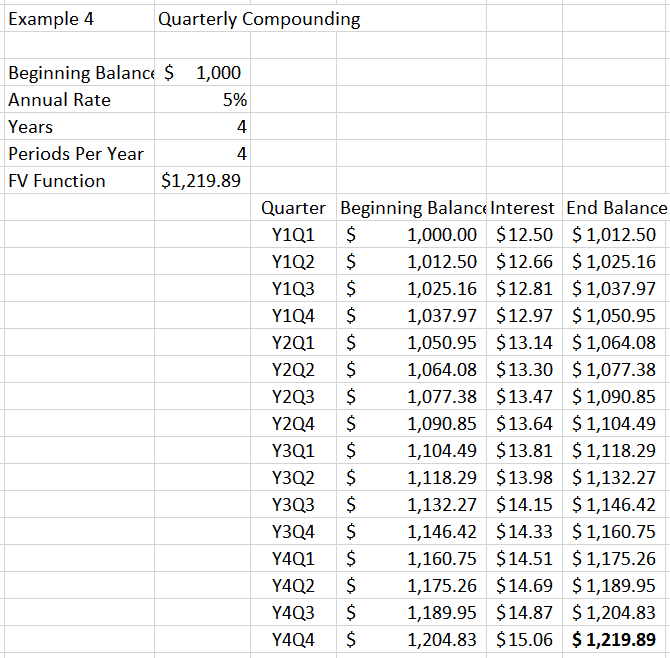#### Monthly Compounding

Using the same example from above and compounding monthly, the future value is \$1,220.90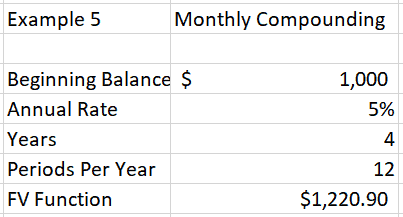#### Daily Compounding

Using the same example from above and compounding daily, the future value is \$1,221.39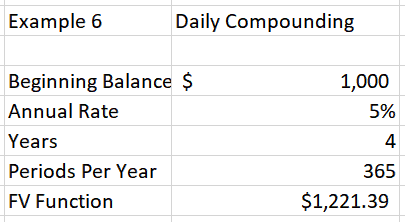When you’re in the wealth-building stage, you’re likely adding more funds to your investments regularly.

It’s straightforward to add a regular investment contribution to the compound interest calculation in both the FV formula and spreadsheet tables.

Here’s a view of the input values and FV function for adding a \$100 monthly contribution to the equation.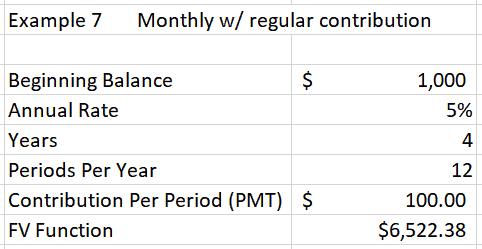Next is a view of the first eight rows (and the last row, 48) of the expanded table to accommodate the regular contribution.

Calculate the interest for the month before adding the contribution.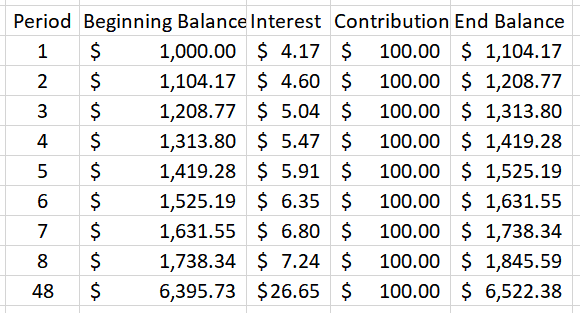### Where the Magic Happens — Exponential Growth

So far, we’ve only been looking at a four-year period. The magic of compound interest happens when you space the model out over decades.

That’s why the most common piece of investing advice you hear is to get started early. The longer your money is invested, the wealthier you will become.

Time, interest rate, and contributions each play a role. At the tail end of the timeline, wealth accumulation accelerates.

In this next example, we use the same variables, but we’ll extend the model to 40 years instead of four. Example #8 uses annual compounding and a \$100 monthly contribution (calculated annually).

At a 5% interest rate, here’s what we get: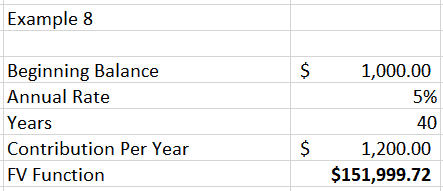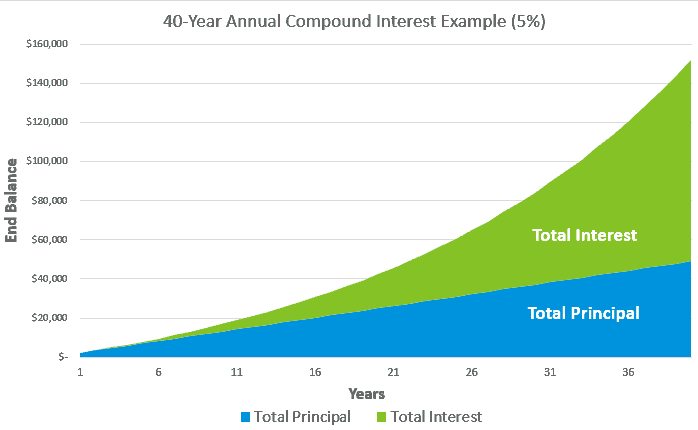Over the 40 years, the investor contributes a total of \$49,000 and ends up with about \$152,000. That’s \$103,000 of earned interest. Not bad.

Looking at the above chart, the principal makes up nearly all of the balance for the first five years. But over time, interest becomes the more powerful wealth-building engine.

What if we increase the rate of return to 10% to keep all the other parameters the same?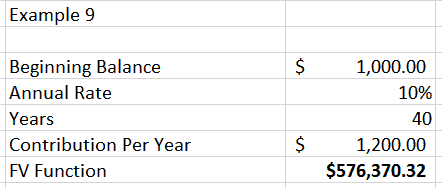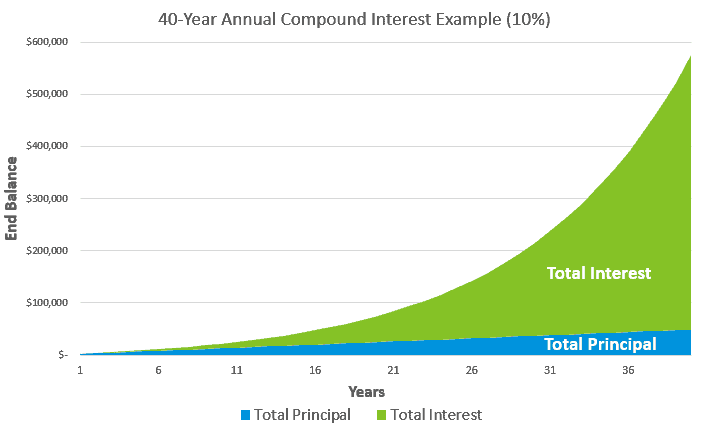The principal amount invested is exactly the same at \$49,000 (the blue shaded areas represent an equal value on both charts).

However, the investor ends up with about \$576,000. The total interest earned in this scenario is \$527,370.32 — much higher.

That’s why investors are so obsessed with achieving high rates of return.

Finally, we change from annual contributions and compounding, to monthly. The other parameters stay the same.

In this last scenario, the investor contributes \$100 per month to an investment portfolio, and over the 40 years, we expect the investor can earn 10% returns.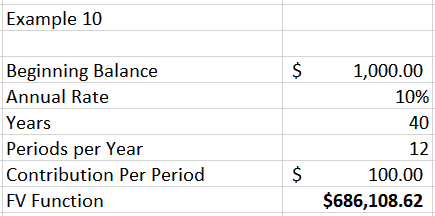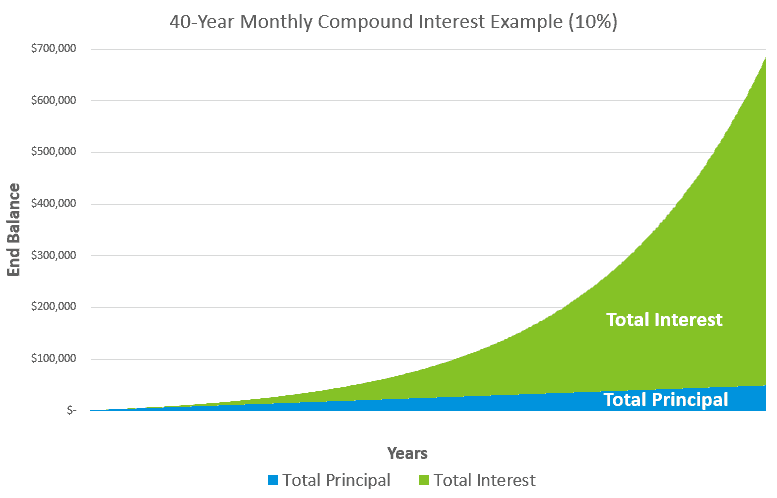By contributing the same annual today, but now every month, and by adjusting the compounding calculator to monthly, the future portfolio value is \$686,000.

That’s \$110,000 more than the previous example with annual contribution and compounding.

That’s the magic of compound interest and exponential growth.

## Conclusion – Compound Interest Examples

It’s not the math that’s hard. Building an initial wealth foundation takes time and sacrifice — the magic of compounding doesn’t happen overnight.

Most people don’t have the patience and don’t like the sacrifice part.

Many spending and financial decisions I make are after consideration of the opportunity cost. Should I buy that thing, or invest the money to let it compound over time?

Compound interest is fundamental to my business too.

The original idea for this blog was to help me earn more money to accelerate my wealth trajectory, investing profits into income-producing assets to add fuel to the compounding fire.

The business name under which I operate this blog is derived from the word “anatocism,” which means compound interest.

Smart investors do four things:

1. Contribute to retirement and investment accounts at least every month
2. Have a long-term investment horizon
3. Diversify to reduce risk
4. Aim to maximize returns based on risk tolerance

You are in total control of items one, two, and three. Doing those three things will get you 75% of the way to being wealthy if you’re patient and stick to a plan.

Item number four is tricky, which is perhaps why investors place so much emphasis on returns.

Example 10 above is a realistic scenario for anyone with a long-term horizon (20+ years). Enter your own numbers to see how the future value of your portfolio looks.

Though higher returns steepen curve toward the end of the chart, you won’t get far without focusing on increasing the input first.

That’s why income and savings rates are more important than investment returns for newer investors.

Later-stage investors face the challenge of balancing risk tolerance and capital preservation with maintaining investment returns. A proper balance enables the continuation of compound interest when it matters most.

Favorite tools and investment services right now:

Sure Dividend — A reliable stock newsletter for DIY retirement investors. (review)

Fundrise — Simple real estate and venture capital investing for as little as \$10. (review)

NewRetirement — Spreadsheets are insufficient. Get serious about planning for retirement. (review)

M1 Finance — A top online broker for long-term investors and dividend reinvestment. (review)

This site uses Akismet to reduce spam. Learn how your comment data is processed.

1. ward139 says:

I really enjoyed the article. Great content and great graphs! When highlighting the effect of compounding interest with my brother, I referred to it as the ‘Splendor’ — a boardgame I like to play with him and his family. Sustained discipline over time is not a glamorous selling point though.

A future post on how compound interest intersects w/ overpaying the mortgage and whether that overpayment acts as a bond for the remaining life of the loan and how that relates to a portfolio’s investment allocation would be something I’d look forward to hearing your thoughts on…as well as the opportunity costs of paying down low interest loan over investing.

I appreciate the encouragement to steadily invest in retirement and investment accounts. I have not steadily invested in my taxable accounts. I’ll have to reevaluate my participation. Thanks for another great post.

2. ATD says:

Thanks for the great post and nice to have the spreadsheet to play with!

One thing that I’ve always wondered is this: For a retirement account that is 100% equities, aren’t you only going to get compound interest on dividends received (about 2% for a S&P500 tracker)? If you invest regularly you are buying more units, but it’s not compounding.

3. Matt says:

Hi there, I am looking for a calculator that increases the contribution to a 457 account annually. For instance, let’s say year one you invest 1% of your gross salary. Year two you invest 2% and so on for 15 years so that every year you increase that contribution by 1%. My intent is to invest some of my yearly raises to make up ground. Is there a calculator or spreadsheet that can do this and figure the compound interest? I have done the math by hand and it looks promising but I would like to check my math against a calculator. Thanks!